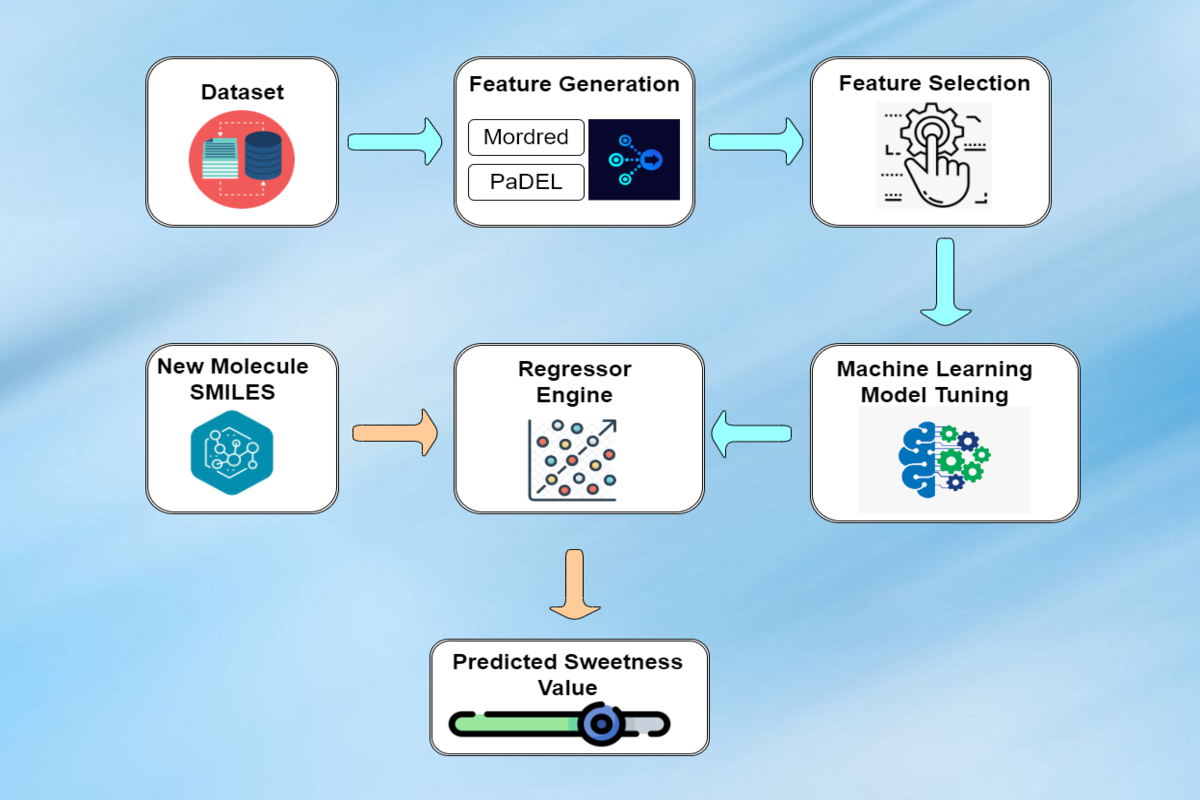Obtaining a sufficient amount of experimentally validated data is one of the most difficult challenges in bioinformatics. A total of 811 sweet molecules were extracted from distinct sources with sweetness value ranging from 0.2 to 22,500,000. 164 redundant molecules were omitted using pybel library to obtain the most extensive dataset of 647 sweet molecules with molecular weight ranging from 122.14 to 1287.62 Daltons, named SweetpredDB. Due to the wide range of sweetness values, we considered the log sweetness (logSw), which ranges from -0.69 to 7.35.

After successful data collection, Molecular features were generated using PaDel and Mordred software comprised of structural, thermodynamical, atomic, topological, chemical, etc. properties of each compound.

Relevant features were selected using Variance-Threshold method (remove features having low variance) and the correlation-based method (remove features correlating ≥ 0.7). SelectKBest feature selection algorithm were implemented which enhances the model accuracy to predict the sweetness of small molecules.

Several regression models, namely Gradient Boost Regressor (GBR), Random Forest Regressor (RF), Multilayer Perceptron Regressor (MLP), Adaboost Regressor (AR), Lasso Regressor (LR), Ridge Regressor (RR), and XGBoost Regressor (XGB) were implemented to predict the sweetness of compounds. The model's efficiency in predicting a molecule's sweetness was obtained using correlation coefficient (R), root mean square error (RMSE), and mean absolute error (MAE). Gradient Boost and Random Forest Regressor outperform other models with correlation coefficient and root mean square error of 0.94, 0.23, and 0.92, 0.28, respectively.